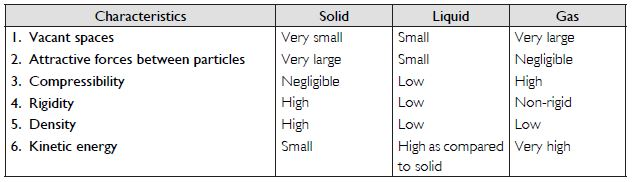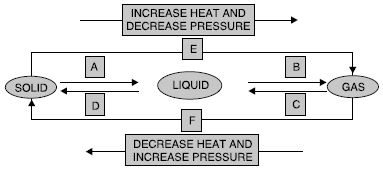# NCERT Solutions for Class 9 Science Chapter 1 - Matter in our surroundings

##### Question 1:

Which of the following are matter?
Chair, air, love, smell, hate, almonds, thought, cold, cold drink, smell of perfume

Chair, air, almonds, cold drink and smell of perfume.

##### Question 2:

Give reasons for the following observation:
The smell of hot sizzling food reaches you several metres away, but to get the smell from cold food you have to go close.

This is because the rate of diffusion of a gas increases with the increase in temperature. In hot food, the rate of diffusion is larger than that in cold food.

##### Question 3:

A diver is able to cut through water in a swimming pool, which property of matter does this observation show?

The diver is able to cut through water in a swimming pool because in water the attractive forces between the molecules are small.

##### Question 4:

What are the characteristics of the particles of matter?

The characteristics of the particles of matter are:

1. The have vacant spaces between them.
2. The are always in motion.
3. There are attractive forces between particles of matter.
##### Question 5:

The mass per unit volume of a substance is called density (density = mass/volume). Arrange the following in order of increasing density:
air, exhaust from chimneys, honey, water, chalk, cotton and iron.

The increasing order of density is:
(air < exhaust from chimney < cotton < water < honey < chalk < iron).

##### Question 6:

(a) Tabulate the differences in the characteristics of states of matter.
(b) Comment upon the following:
rigidity, compressibility, fluidity, filling a gas container, shape, kinetic energy and density.

(a) Differences in the characteristics of states of matter:(b) Rigidity. It is the property by virtue of which a substance can retain its shape when a force is applied to it. Solids possess the property of rigidity.
Compressibility. The property by virtue of which the volume of a substance can be decreased by applying force or pressure on it is known as compressibility. Gases have high compressibility because of large vacant spaces between the molecules of a gas. Fluidity. It is the property by virtue of which the molecules of one substance can flow from one point to another. Liquids and gases possess fluidity.
Filling a gas container. A gas fills a container because there are negligible attractive forces between the molecules of a gas and the molecules move with very high velocities in all possible directions. Shape. It is the definite geometrical arrangement of constituent particles of a substance. Solids have definite shapes.
Kinetic energy. It is the energy possessed by a particle due to its motion.
Density. Density of a substance is the mass per unit volume. Its units are g/cm3 or kg/m3.

##### Question 7:

Give reasons:

1. A gas fills completely the vessel in which it is kept.
2. A gas exerts pressure on the walls of the container.
3. A wooden table should be called a solid.
4. We can easily move our hand in air but to do the same through a solid block of wood we need a karate expert.

(a) This is so because in a gas the attractive forces between the particles are negligible and molecules of a gas move with very high speeds in all possible directions.
(b) This is due to the bombardment of the molecules of a gas against the walls of the container.
(c) This is because it has a definite mass, volume and shape.
(d) This is because in air, the attractive forces between the particles are negligible but in a solid like block of wood, there are large attractive forces between the constituent particles.

##### Question 8:

Liquids generally have lower density as compared to solids. But you must have observed that ice floats on water. Find out why.

Ice floats over water because ice has lower density than liquid water. This is because for a given mass, ice has larger volume than liquid water.

##### Question 9:

Convert the following temperatures to celsius scale:
(a) 300 K
(b) 573 K

(a) 300 K = 300 - 273 = 27$°$C
(b) 573 K = 573 - 273 = 300$°$C

##### Question 10:

What is the physical state of water at:
(a) 250$°$C
(b) 100$°$C

(a) Vapour
(b) Vapour

##### Question 11:

For any substance, why does the temperature remain constant during the change of state?

This is because the supplied heat is used to overcome the attractive forces between the particles.

##### Question 12:

Suggest a method to liquefy atmospheric gases.

The atmospheric gases can be liquefied by cooling the gases under high pressure.

##### Question 13:

Why does a desert cooler cool better on a hot dry day?

This is because on a hot dry day, the evaporation of water is faster due to higher temperature and lower humidity.

##### Question 14:

How does the water kept in an earthen pot (matka) become cool during summer?

This is because water can move out from the pores of earthen pot (matka) and it evaporates. Due to evaporation, water in the earthern pot cools down.

##### Question 15:

Why does our palm feel cold when we put some acetone or petrol or perfume on it?

This is because the particles of acetone or petrol or perfume (being low boiling liquids) gain energy from our palm and the surroundings and thus evaporate to cause cooling.

##### Question 16:

Why are we able to sip hot tea or milk faster from a saucer rather than a cup?

Because a saucer provides more surface area than a cup for evaporation of the liquid. It causes more cooling effect.

##### Question 17:

What type of clothes should we wear in summer?

We should wear light coloured cotton clothes in summer.

##### Question 18:

Convert the following temperatures to the Celsius scale.
(a) 293 K
(b) 470 K

(a) 293 K = 293 – 273 = 20$°$C (b) 470 K = 470 – 273 = 197$°$C

##### Question 19:

Convert the following temperatures to the Kelvin scale.
(a) 25$°$C
(b) 373$°$C

(a) 25$°$C = 25 + 273 = 298 K
(b) 373$°$C = 373 + 273 = 646 K

##### Question 20:

Give reason for the following observations.
(a) Naphthalene balls disappear with time without leaving any solid.
(b) We can get the smell of perfume sitting several

(a) This is because naphthalene sublimes i.e. it directly changes into vapour without melting.
(b) This is because the molecules of perfume are moving with very high speed (diffusion) in all the directions.

##### Question 21:

Arrange the following substances in increasing order of forces of attraction between the particles: water, sugar, oxygen.

The increasing order of intermolecular forces of attraction is oxygen < water < sugar.

##### Question 22:

What is the physical state of water at:
(a) 25$°$C
(b) 0$°$C
(c) 100$°$C

(a) At 25$°$C, water is liquid.
(b) At 0$°$C, water is solid (lce).
(c) At 100$°$C, water is vapour (steam).

##### Question 23:

Give two reasons to justify —
(a) water at room temperature is a liquid.
(b) an iron almirah is a solid at room temperature.

(a) At room temperature water is liquid because the attractive forces between the molecules of water are small and can move from one point to another.
(b) An iron almirah is a solid because the particles are held together by strong intermolecular attractive forces and these particles are very close to each other.

##### Question 24:

Why is ice at 273 K more effective in cooling than water at the same temperature?

Ice at 273 K is more effective in cooling than water at the same temperature because in ice, the molecules have lower energy as compared to the particles in the liquid water at the same temperature (273 K) and require latent heat of fusion for melting.

##### Question 25:

What produces more severe burns, boiling water or steam?